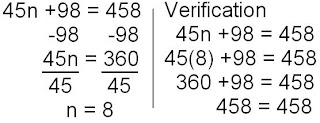### Scribe Post for January 26, 2009

Monday, January 26, 2009
Today in class, we went over some word problems. The word problems that we did, are located on pages 44, 45, and 46 of your purple booklet. In this scribe I will show 3 word problems, and I will explain a little bit of each, so that it makes it easy for you to do.

When you have a word problem, you must answer the word problem with the last sentence from the problem. For example: If the last sentence of a question is "Find the number", then your answer would be "The number is ____". Something like that. There is always numbers in the questions, you just have to find them. Try looking for the variable first, and then the constant. I find it easier for me.

BLUE = The variable in word problem
GREEN = The constant in word problem
RED = The operation (What you're doing to the constant.)
PINK = The answer in word problem
____________________________________

First Question :
Page 44, #15

The sum of eight times a number and fifteen is seven. Find the number.
The number is __
Bring down all of the numbers that you could identify, and this question should look like this.
8n + 15 = 7The number is -1

REMEMBER
- always end your word problem with a sentence solving the question.
For this question, the sentence is right above.

Second Question
Page 45, Letter N

Mo is thinking of a number. Eleven more than one third of the number is -1. Find Mo's number.
n/3 + 11 = -1Mo's number is -36.

Third Question
Page 45, Letter T

NOTE - Some questions will make it harder for you to find the numbers, but just relax, and take time to find them all.

Mr. Piper's plumbing needed repairs. The plumber charged \$98 for parts plus \$45 per hour for labor. If the bill totaled \$458, how many hours of labor were required?
45h + 98 = 4588 hours of labor was required.
_____________________________________

Anyways, thanks for reading. And sorry for doing such a bad job, I had to rush most of these.
The next scribe is JAYZIE.
COMMENT!